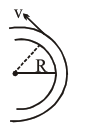# A modern grand-prix`
Question:

A modern grand-prix racing car of mass $m$ is travelling on a flat track in a circular arc of radius $R$ with a speed $v$. If the coefficient of static friction between the tyres and the track is $\mu_{\mathrm{s}}$, then the magnitude of negative lift $\mathrm{F}_{\mathrm{L}}$ acting downwards on the car is :

(Assume forces on the four tyres are identical and $g=$ acceleration due to gravity)1. $\mathrm{m}\left(\frac{\mathrm{v}^{2}}{\mu_{\mathrm{s}} \mathrm{R}}+\mathrm{g}\right)$

2. $m\left(\frac{v^{2}}{\mu_{s} R}-g\right)$

3. $m\left(g-\frac{v^{2}}{\mu_{s} R}\right)$

4. $-m\left(g+\frac{v^{2}}{\mu_{s} R}\right)$

Correct Option: , 2

Solution:

$\mu_{\mathrm{s}} \mathrm{N}=\frac{\mathrm{mv}^{2}}{\mathrm{R}}$

$\mathrm{N}=\frac{\mathrm{mv}^{2}}{\mu_{\mathrm{s}} \mathrm{R}}=\mathrm{mg}+\mathrm{F}_{\mathrm{L}}$

$\mathrm{F}_{\mathrm{L}}=\frac{\mathrm{mv}^{2}}{\mu_{\mathrm{s}} \mathrm{R}}-\mathrm{mg}$